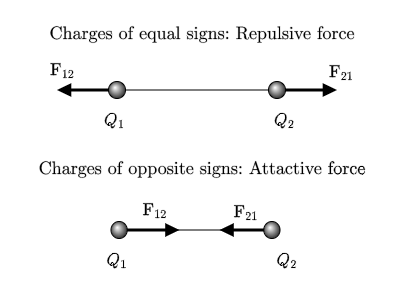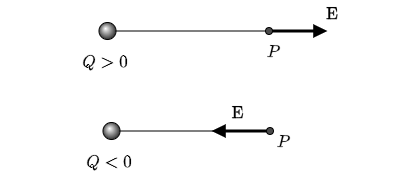# Three positive charges, A, B, and C, of magnitudes 3 micro C, 2 micro C, and 2 micro C...

## Question:

Three positive charges, A, B, and C, of magnitudes {eq}\rm 3 \ \mu C, 2 \ \mu C, {/eq} and {eq}\rm 2 \ \mu C {/eq} respectively, are located at the corners of an equilateral triangle of side 0.2 m.

a. Find the magnitude of the force on each charge.

b. Find the magnitude of the electric field at the center of the triangle.

## Coulomb's Law

We use in this answer the Coulomb's law to determine properties of a system of electrical charges.

Coulomb's law gives the force between two charges at a distance {eq}d {/eq} apart and its magnitude is given by

$$F = k \dfrac{ Q_{1} Q_{2} }{ d^{2} } \, \, ,$$

where $$k = 9.0 \times 10^{9} \, \text{N} \cdot \text{m}^{2} \cdot \text{C}^{-2} \, \, .$$

The charges {eq}Q_1 {/eq} and {eq}Q_2 {/eq} may be positive or negative. The force is attractive for charges of opposite signs and repulsive for charges of equal signs.The electric force obeys the superposition principle, which means that the resultant force on a charge due to a collection of charges is the vectorial sum of the forces due to each charge.

\begin{align} \mathbf{F}_{R} &= \sum_{i=1}^{N} \mathbf{F}_{i} \\ \\ &= \mathbf{F}_{1} + \mathbf{F}_{2} + \cdots + \mathbf{F}_{N} \, \, . \end{align}

The magnitude of the electric field produced by a charge {eq}Q {/eq} at a point {eq}P {/eq} at a distance {eq}r {/eq} away has magnitude given by

$$E = k \dfrac{ Q }{ r^{2} } \, \, ,$$

and the direction of the electric field vector points away from positive charges and into negative ones.The electric field also obeys the superposition principle. The electric field due to a collection of charges at a point of space is the vector sum of the fields produced by each of the charges,

\begin{align} \mathbf{E}_{R} &= \sum_{i=1}^{N} \mathbf{E}_{i} \\ \\ &= \mathbf{E}_{1} + \mathbf{E}_{2} + \cdots + \mathbf{E}_{N} \, \, . \end{align}

Become a Study.com member to unlock this answer! Create your account

The charges are {eq}Q_{A} = 3 \mu\text{C} {/eq}, {eq}Q_{B} = 2 \mu\text{C} {/eq} and {eq}Q_{C} = 2 \mu\text{C} {/eq}. The distance between then is...